# Golang Struct: The Complete Guide

Go struct can be used where it makes sense to group data into a single unit rather than maintaining each as a separate type.

Any real-world entity with a set of properties can be represented using a struct. Structs are useful for grouping data together to create records.

If you’re coming from an object-oriented programming background, you can think of a struct as a lightweight class that supports the composition but not an inheritance.

## Golang Struct

Golang struct is a user-defined type that represents the collection of named fields/properties. Go struct is used to group related data to form a single unit.

## How to declare struct in Golang

To declare a struct in Golang, use the keyword type, then a name for the new struct, and finally, the keyword struct. For instance, a student has firstName, lastName, and age.

It makes sense to group these three fields into a single structure student.

```type Student struct {
firstName string
lastName  string
age       int
}```

The above snippet declares a structure type Student with fields first_name, last_name, and age.

The structure can also be made more compact by declaring the fields that belong to the same type in a single line followed by a type name.

In the above struct program, firstName and lastName belong to the same type string, age as an integer, and hence the struct can be rewritten as the following code.

```type Student struct {
firstName, lastName string
age int
}```

## Creating named structures in Go

Let’s define a named structure Student using a simple program.

```// hello.go

package main

import "fmt"

// Student structure
type Student struct {
firstName, lastName string
age                 int
}

func main() {

// creating first student structure using field names
stud := Student{
firstName: "Krunal",
lastName:  "Lathiya",
age:       26,
}

//creating second structure without using field names
stud2 := Student{"Ankit", "Lathiya", 25}

fmt.Println("Student 1", stud)
fmt.Println("Student 2", stud2)
}```

In the above program, we create a named struct Student. Then stud structure is defined by specifying the value for each field name. It is not necessary that the order of the field names should be the same as that while declaring the structure type.

In the above program, stud2 is defined by omitting the property names.

In that case, it is necessary to maintain an order of properties to be the same as specified in the structure declaration.

Finally, we have printed both the structs into the terminal console. See the below output.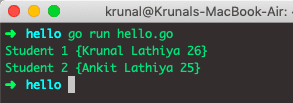## Creating anonymous structs in Go

See the below code example.

```// hello.go

package main

import "fmt"

func main() {
stud3 := struct {
firstName, lastName string
age                 int
}{
firstName: "Rushabh",
lastName:  "Rupani",
age:       27,
}

fmt.Println("Student 3", stud3)
}
```

In the above program, an anonymous structure variable stud3 is defined. As we have already mentioned, the above structure is called anonymous because it only creates a new struct variable stud3 and does not define any new struct type.

The output is the following.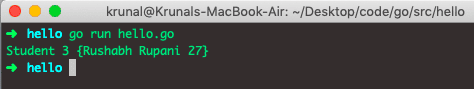## Zero value of a structure in Go

When the struct is defined and not explicitly initialized with any value, the properties of a struct are assigned their zero values by default. See the below code example.

```// hello.go

package main

import (
"fmt"
)

// Student struct
type Student struct {
firstName, lastName string
age                 int
}

func main() {
var stud4 Student //zero valued structure
fmt.Println("Student 4", stud4)
}
```

The above program defines stud4 but is not initialized with any value. Hence the firstName and lastName are assigned the zero values of string which is ” “, and age is assigned the zero values of int, which is 0.

See the below output.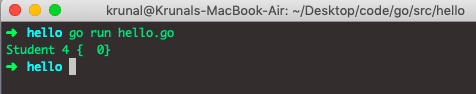## Accessing individual fields of a struct

The dot (.) operator is used to access the different fields of a structure.

See the below code.

```// hello.go

package main

import "fmt"

// Student structure
type Student struct {
firstName, lastName string
age                 int
}

func main() {
stud5 := Student{"Robert", "Downey", 55}
fmt.Println("First Name:", stud5.firstName)
fmt.Println("Last Name:", stud5.lastName)
fmt.Println("Age:", stud5.age)
}
```

See the below output.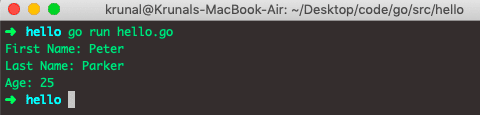It is also possible to create a zero struct and then assign the values to its fields later.

See the below code.

```// hello.go

package main

import "fmt"

// Student strucutre
type Student struct {
firstName, lastName string
age                 int
}

func main() {
var stud6 Student
stud6.firstName = "Ken"
stud6.age = 30
fmt.Println("First Name:", stud6.firstName)
fmt.Println("Last Name:", stud6.lastName)
fmt.Println("Age:", stud6.age)
}
```

In the above code, we have first created a zero struct and then assigned the different fields to different values and then printed them in the console.

See the below output.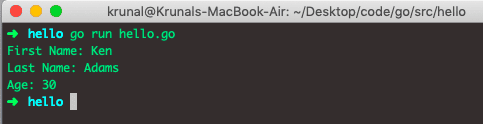## Pointers to a struct

It is also possible to create the pointers to the struct. See the below code.

```// hello.go

package main

import "fmt"

// Student structure
type Student struct {
firstName, lastName string
age                 int
}

func main() {
fmt.Println("First Name:", (*stud7).firstName)
fmt.Println("Last Name:", (*stud7).lastName)
fmt.Println("Age:", (*stud7).age)
}
```

The stud7 in the above program is a pointer to the Student struct. The (*stud7).firstName is the syntax to access the firstName field of the stud7 struct. See the below output.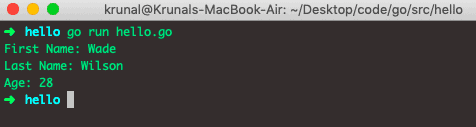The Golang gives us the option to use stud7.firstName instead of the explicit dereference (*stud7).firstName to access the firstName field.

## Anonymous fields in Golang

It is possible to create the structs with fields that contain only the type without the field name. This type of field is called an anonymous field. The snippet below creates a struct Student with two anonymous fields, string, and int.

```type Student struct {
string
int
}```

Let’s write a program using anonymous fields.

```// hello.go

package main

import "fmt"

// Student struct
type Student struct {
string
int
}

func main() {
s1 := Student{"Krunal", 26}
fmt.Println(s1)
}
```

The Student is a struct with two anonymous fields in the above program. The s1 := Student{“Krunal”, 26}  defines a variable of type Student. Even though an anonymous field does not have a name, by default, the name of an anonymous field is the name of its type.

For example, in the case of the Student struct above, although the fields are anonymous, they take the firstName of the type of the fields by default. So Student struct has two fields with name string and int.

The output is the following.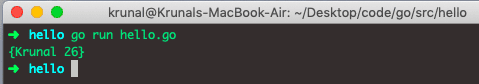## Nested structs in Golang

It is possible that a struct contains the field, which is the struct. This kind of struct is called a nested struct. See the below code example.

```// hello.go

package main

import "fmt"

city, state string
}

// Student struct
type Student struct {
name    string
age     int
}

func main() {
var s1 Student
s1.name = "Krunal"
s1.age = 26
city:  "Rajkot",
state: "Gujarat",
}
fmt.Println(s1)
}
```

The Student struct in the above program has a field address, which is a struct. This program outputs the following.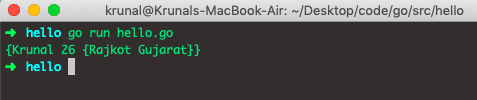## Struct Methods in Go

In the below code example, we will define a struct called Rectangle, and then we calculate the area of the rectangle by determining some methods.

```// hello.go

package main

import (
"fmt"
"math"
)

// Rectangle Struct
type Rectangle struct {
x1, y1, x2, y2 float64
}

func distance(x1, y1, x2, y2 float64) float64 {
a := x2 - x1
b := y2 - y1
return math.Sqrt(a*a + b*b)
}

func (r *Rectangle) area() float64 {
l := distance(r.x1, r.y1, r.x1, r.y2)
w := distance(r.x1, r.y1, r.x2, r.y1)
return l * w
}

func main() {
r := Rectangle{0, 0, 19, 21}
fmt.Println("The area of Rectangle is:", r.area())
}
```

In the above code, we have defined two methods.

1. distance
2. area

We need a distance to calculate the area. Finally, we have called the area method from the main() method. It gives the output. See the below output.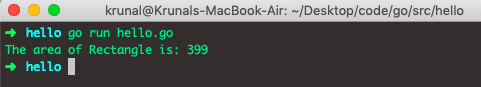#### Creating a struct and obtaining the pointer to it using the built-in new() function

You can also use the new() function to create the instance of the struct.

A new() function allocates enough memory to fit all the struct properties, sets each to their zero value, and returns the pointer to the newly allocated struct. See the below code.

```// hello.go

package main

import "fmt"

// Student struct
type Student struct {
name string
age  int
}

func main() {
s2 := new(Student)
s2.name = "Krunal"
s2.age = 26

fmt.Println(s2)
}
```

See the below output.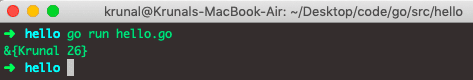## Struct Equality in Go

Two struct variables are counted as equal if all their corresponding fields are equal. See the below code.

```// hello.go

package main

import "fmt"

// Student struct
type Student struct {
name string
age  int
}

func main() {
s1 := Student{"Krunal", 26}
s2 := Student{"Krunal", 26}

if s1 == s2 {
fmt.Println("Student s1 and s2 are equal.")
} else {
fmt.Println("Student s1 and s2 are not equal.")
}
}
```

In the above code, both the s1 and s2 are equal with the values, which is why both the structs are identical.

If any struct values differ, then they will not equal each other.

## Conclusion

In this post, we have seen how to initialize the struct, create an anonymous struct, properties of structs, methods of structs, pointers in a struct, etc.

That’s it.

## Recommended Posts

Golang Function

Golang Slice

Go Slice Append

Go For Loop

Go Custom Type Declarations

Categories Go

This site uses Akismet to reduce spam. Learn how your comment data is processed.